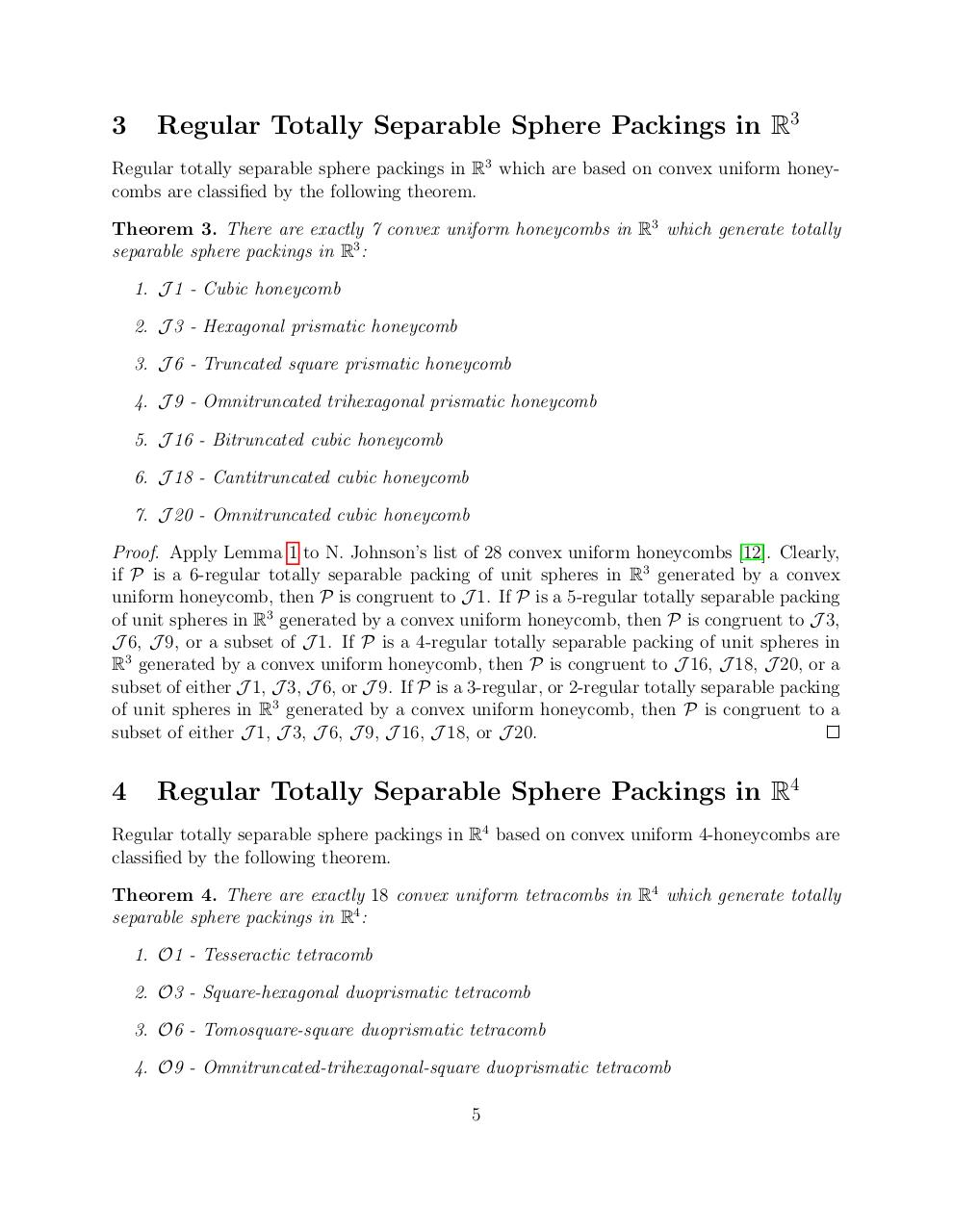# Regular Totally Separable Sphere Packings arXiv.pdfPage 1 2 3 4 5 6 7 8 9 10

#### Text preview

3

Regular Totally Separable Sphere Packings in R3

Regular totally separable sphere packings in R3 which are based on convex uniform honeycombs are classified by the following theorem.
Theorem 3. There are exactly 7 convex uniform honeycombs in R3 which generate totally
separable sphere packings in R3 :
1. J 1 - Cubic honeycomb
2. J 3 - Hexagonal prismatic honeycomb
3. J 6 - Truncated square prismatic honeycomb
4. J 9 - Omnitruncated trihexagonal prismatic honeycomb
5. J 16 - Bitruncated cubic honeycomb
6. J 18 - Cantitruncated cubic honeycomb
7. J 20 - Omnitruncated cubic honeycomb
Proof. Apply Lemma 1 to N. Johnson’s list of 28 convex uniform honeycombs . Clearly,
if P is a 6-regular totally separable packing of unit spheres in R3 generated by a convex
uniform honeycomb, then P is congruent to J 1. If P is a 5-regular totally separable packing
of unit spheres in R3 generated by a convex uniform honeycomb, then P is congruent to J 3,
J 6, J 9, or a subset of J 1. If P is a 4-regular totally separable packing of unit spheres in
R3 generated by a convex uniform honeycomb, then P is congruent to J 16, J 18, J 20, or a
subset of either J 1, J 3, J 6, or J 9. If P is a 3-regular, or 2-regular totally separable packing
of unit spheres in R3 generated by a convex uniform honeycomb, then P is congruent to a
subset of either J 1, J 3, J 6, J 9, J 16, J 18, or J 20.

4

Regular Totally Separable Sphere Packings in R4

Regular totally separable sphere packings in R4 based on convex uniform 4-honeycombs are
classified by the following theorem.
Theorem 4. There are exactly 18 convex uniform tetracombs in R4 which generate totally
separable sphere packings in R4 :
1. O1 - Tesseractic tetracomb
2. O3 - Square-hexagonal duoprismatic tetracomb
3. O6 - Tomosquare-square duoprismatic tetracomb
4. O9 - Omnitruncated-trihexagonal-square duoprismatic tetracomb
5